# Acceleration

AccelerationIn the absence of air resistance and thus terminal velocity, a falling ball would continue to accelerate.
Common symbols
a
SI unit m/s2, m·s−2, m s−2

Acceleration, in physics, is the rate of change of velocity of an object with respect to time. An object's acceleration is the net result of any and all forces acting on the object, as described by Newton's Second Law. The SI unit for acceleration is metre per second squared (m s−2). Accelerations are vector quantities (they have magnitude and direction) and add according to the parallelogram law. As a vector, the calculated net force is equal to the product of the object's mass (a scalar quantity) and its acceleration.

For example, when a car starts from a standstill (zero relative velocity) and travels in a straight line at increasing speeds, it is accelerating in the direction of travel. If the car turns, there is an acceleration toward the new direction. In this example, we can call the forward acceleration of the car a "linear acceleration", which passengers in the car might experience as a force pushing them back into their seats. When changing direction, we might call this "non-linear acceleration", which passengers might experience as a sideways force. If the speed of the car decreases, this is an acceleration in the opposite direction from the direction of the vehicle, sometimes called deceleration. Passengers may experience deceleration as a force lifting them forwards. Mathematically, there is no separate formula for deceleration: both are changes in velocity. Each of these accelerations (linear, non-linear, deceleration) might be felt by passengers until their velocity (speed and direction) matches that of the car.

## Definition and properties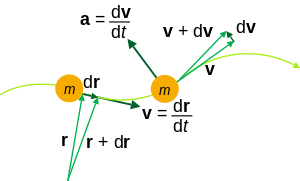Kinematic quantities of a classical particle: mass m, position r, velocity v, acceleration a.

### Average acceleration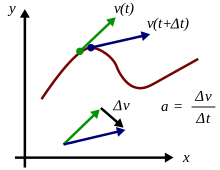Acceleration is the rate of change of velocity. At any point on a trajectory, the magnitude of the acceleration is given by the rate of change of velocity in both magnitude and direction at that point. The true acceleration at time t is found in the limit as time interval Δt → 0 of Δv/Δt

An object's average acceleration over a period of time is its change in velocitydivided by the duration of the period. Mathematically,### Instantaneous acceleration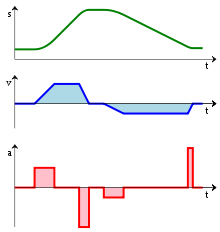From bottom to top:
• an acceleration function a(t);
• the integral of the acceleration is the velocity function v(t);
• and the integral of the velocity is the distance function s(t).

Instantaneous acceleration, meanwhile, is the limit of the average acceleration over an infinitesimal interval of time. In the terms of calculus, instantaneous acceleration is the derivative of the velocity vector with respect to time:(Here and elsewhere, if motion is in a straight line, vector quantities can be substituted by scalars in the equations.)

It can be seen that the integral of the acceleration function a(t) is the velocity function v(t); that is, the area under the curve of an acceleration vs. time (a vs. t) graph corresponds to velocity.As acceleration is defined as the derivative of velocity, v, with respect to time t and velocity is defined as the derivative of position, x, with respect to time, acceleration can be thought of as the second derivative of x with respect to t:### Units

Acceleration has the dimensions of velocity (L/T) divided by time, i.e. L.T−2. The SI unit of acceleration is the metre per second squared (m s−2); or "metre per second per second", as the velocity in metres per second changes by the acceleration value, every second.

### Other forms

An object moving in a circular motionsuch as a satellite orbiting the Earthis accelerating due to the change of direction of motion, although its speed may be constant. In this case it is said to be undergoing centripetal (directed towards the center) acceleration.

Proper acceleration, the acceleration of a body relative to a free-fall condition, is measured by an instrument called an accelerometer.

In classical mechanics, for a body with constant mass, the (vector) acceleration of the body's center of mass is proportional to the net force vector (i.e. sum of all forces) acting on it (Newton's second law):where F is the net force acting on the body, m is the mass of the body, and a is the center-of-mass acceleration. As speeds approach the speed of light, relativistic effects become increasingly large.

## Tangential and centripetal acceleration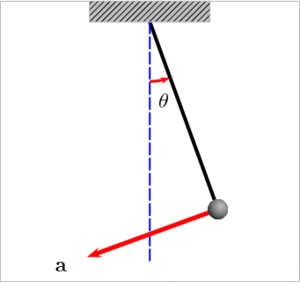An oscillating pendulum, with velocity and acceleration marked. It experiences both tangential and centripetal acceleration.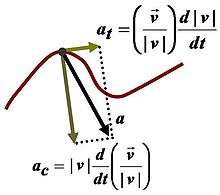Components of acceleration for a curved motion. The tangential component at is due to the change in speed of traversal, and points along the curve in the direction of the velocity vector (or in the opposite direction). The normal component (also called centripetal component for circular motion) ac is due to the change in direction of the velocity vector and is normal to the trajectory, pointing toward the center of curvature of the path.

The velocity of a particle moving on a curved path as a function of time can be written as:with v(t) equal to the speed of travel along the path, anda unit vector tangent to the path pointing in the direction of motion at the chosen moment in time. Taking into account both the changing speed v(t) and the changing direction of ut, the acceleration of a particle moving on a curved path can be written using the chain rule of differentiation for the product of two functions of time as:where un is the unit (inward) normal vector to the particle's trajectory (also called the principal normal), and r is its instantaneous radius of curvature based upon the osculating circle at time t. These components are called the tangential acceleration and the normal or radial acceleration (or centripetal acceleration in circular motion, see also circular motion and centripetal force).

Geometrical analysis of three-dimensional space curves, which explains tangent, (principal) normal and binormal, is described by the Frenet–Serret formulas.

## Special cases

### Uniform acceleration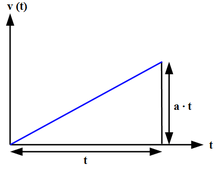Calculation of the speed difference for a uniform acceleration.

Uniform or constant acceleration is a type of motion in which the velocity of an object changes by an equal amount in every equal time period.

A frequently cited example of uniform acceleration is that of an object in free fall in a uniform gravitational field. The acceleration of a falling body in the absence of resistances to motion is dependent only on the gravitational field strength g (also called acceleration due to gravity). By Newton's Second Law the force, F, acting on a body is given by:Because of the simple analytic properties of the case of constant acceleration, there are simple formulas relating the displacement, initial and time-dependent velocities, and acceleration to the time elapsed:where

•is the elapsed time,
•is the initial displacement from the origin,
•is the displacement from the origin at time,
•is the initial velocity,
•is the velocity at time, and
•is the uniform rate of acceleration.

In particular, the motion can be resolved into two orthogonal parts, one of constant velocity and the other according to the above equations. As Galileo showed, the net result is parabolic motion, which describes, e. g., the trajectory of a projectile in a vacuum near the surface of Earth.

### Circular motion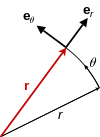Position vector r, always points radially from the origin.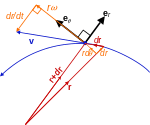Velocity vector v, always tangent to the path of motion.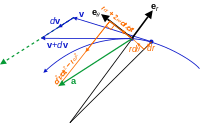Acceleration vector a, not parallel to the radial motion but offset by the angular and Coriolis accelerations, nor tangent to the path but offset by the centripetal and radial accelerations.
Kinematic vectors in plane polar coordinates. Notice the setup is not restricted to 2d space, but a plane in any higher dimension.

Uniform circular motion, that is constant speed along a circular path, is an example of a body experiencing acceleration resulting in velocity of a constant magnitude but change of direction. In this case, because the direction of the object's motion is constantly changing, being tangential to the circle, the object's linear velocity vector also changes, but its speed does not. This acceleration is a radial acceleration since it is always directed toward the centre of the circle and takes the magnitude:whereis the object's linear speed along the circular path. Equivalently, the radial acceleration vector () may be calculated from the object's angular velocity:whereis a vector directed from the centre of the circle and equal in magnitude to the radius. The negative shows that the acceleration vector is directed towards the centre of the circle (opposite to the radius).

The acceleration and the net force acting on a body in uniform circular motion are directed toward the centre of the circle; that is, it is centripetal. Whereas the so-called 'centrifugal force' appearing to act outward on the body is really a pseudo force experienced in the frame of reference of the body in circular motion, due to the body's linear momentum at a tangent to the circle.

With nonuniform circular motion, i.e., the speed along the curved path changes, a transverse acceleration is produced equal to the rate of change of the angular speed around the circle times the radius of the circle. That is,The transverse (or tangential) acceleration is directed at right angles to the radius vector and takes the sign of the angular acceleration ().

## Relation to relativity

### Special relativity

Main article: Special relativity

The special theory of relativity describes the behavior of objects traveling relative to other objects at speeds approaching that of light in a vacuum. Newtonian mechanics is exactly revealed to be an approximation to reality, valid to great accuracy at lower speeds. As the relevant speeds increase toward the speed of light, acceleration no longer follows classical equations.

As speeds approach that of light, the acceleration produced by a given force decreases, becoming infinitesimally small as light speed is approached; an object with mass can approach this speed asymptotically, but never reach it.

### General relativity

Main article: General relativity

Unless the state of motion of an object is known, it is totally impossible to distinguish whether an observed force is due to gravity or to acceleration—gravity and inertial acceleration have identical effects. Albert Einstein called this the principle of equivalence, and said that only observers who feel no force at all—including the force of gravity—are justified in concluding that they are not accelerating.

## Conversions

Conversions between common units of acceleration
Base value (Gal, or cm/s2) (ft/s2) (m/s2) (Standard gravity, g0)
1 Gal, or cm/s2 1 0.0328084 0.01 0.00101972
1 ft/s2 30.4800 1 0.304800 0.0310810
1 m/s2 100 3.28084 1 0.101972
1 g0 980.665 32.1740 9.80665 1

## References

1. Crew, Henry (2008). The Principles of Mechanics. BiblioBazaar, LLC. p. 43. ISBN 0-559-36871-2.
2. Bondi, Hermann (1980). Relativity and Common Sense. Courier Dover Publications. p. 3. ISBN 0-486-24021-5.
3. Lehrman, Robert L. (1998). Physics the Easy Way. Barron's Educational Series. p. 27. ISBN 0-7641-0236-2.
4. Raymond A. Serway; Chris Vuille; Jerry S. Faughn (2008). College Physics, Volume 10. Cengage. p. 32. ISBN 9780495386933.
5. Weisstein, Eric W. "Chain Rule". Wolfram MathWorld. Wolfram Research. Retrieved 2 August 2016.
6. Larry C. Andrews; Ronald L. Phillips (2003). Mathematical Techniques for Engineers and Scientists. SPIE Press. p. 164. ISBN 0-8194-4506-1.
7. Ch V Ramana Murthy; NC Srinivas (2001). Applied Mathematics. New Delhi: S. Chand & Co. p. 337. ISBN 81-219-2082-5.
8. Keith Johnson (2001). Physics for you: revised national curriculum edition for GCSE (4th ed.). Nelson Thornes. p. 135. ISBN 978-0-7487-6236-1.
9. David C. Cassidy; Gerald James Holton; F. James Rutherford (2002). Understanding physics. Birkhäuser. p. 146. ISBN 978-0-387-98756-9.
10. Brian Greene, The Fabric of the Cosmos: Space, Time, and the Texture of Reality, page 67. Vintage ISBN 0-375-72720-5Wikimedia Commons has media related to Acceleration.